# Saturation Behavior: a general relationship described by a simple second-order differential equation

## Abstract

### Background

The numerous natural phenomena that exhibit saturation behavior, e.g., ligand binding and enzyme kinetics, have been approached, to date, via empirical and particular analyses. This paper presents a mechanism-free, and assumption-free, second-order differential equation, designed only to describe a typical relationship between the variables governing these phenomena. It develops a mathematical model for this relation, based solely on the analysis of the typical experimental data plot and its saturation characteristics. Its utility complements the traditional empirical approaches.

### Results

For the general saturation curve, described in terms of its independent (x) and dependent (y) variables, a second-order differential equation is obtained that applies to any saturation phenomena. It shows that the driving factor for the basic saturation behavior is the probability of the interactive site being free, which is described quantitatively. Solving the equation relates the variables in terms of the two empirical constants common to all these phenomena, the initial slope of the data plot and the limiting value at saturation. A first-order differential equation for the slope emerged that led to the concept of the effective binding rate at the active site and its dependence on the calculable probability the interactive site is free. These results are illustrated using specific cases, including ligand binding and enzyme kinetics. This leads to a revised understanding of how to interpret the empirical constants, in terms of the variables pertinent to the phenomenon under study.

### Conclusions

The second-order differential equation revealed the basic underlying relations that describe these saturation phenomena, and the basic mathematical properties of the standard experimental data plot. It was shown how to integrate this differential equation, and define the common basic properties of these phenomena. The results regarding the importance of the slope and the new perspectives on the empirical constants governing the behavior of these phenomena led to an alternative perspective on saturation behavior kinetics. Their essential commonality was revealed by this analysis, based on the second-order differential equation.

## Background

This paper answers the question: is there a general mathematical model common to the numerous natural phenomena that display identical saturation behavior? Examples include ligand binding, enzyme kinetics, facilitated diffusion, predator-prey behavior, bacterial culture growth rate, infection transmission, surface adsorption, and many more. The mathematical model developed here is based on a general second-order differential equation (D.E.), free of empirical constants, that describes the basic relation underlying these saturation phenomena .

A common and productive way to analyze a specific saturation phenomenon uses a model for the proposed mechanism. This leads to an algebraic relation that describes the experimental observations, and helps interpret features of the mechanism. Where the phenomenon involves chemical reactions, for example, the models rely on assumptions about reaction mechanisms, dissociation constants, and mass action rate constants . Note that such mechanisms cannot be proved definitively by standard kinetic studies .

In view of the ubiquity of saturation phenomena, it seems useful to seek one mathematical model that describes all such phenomena. The model presented here relies solely on the basic mathematical properties of the experimentally observed data plot for these phenomena--the independent variable versus the dependent variable. It is free of mechanism and therefore applies uniformly to all these phenomena. The analysis starts with a second-order differential equation, free of constants, that offers a general way of describing them. This equation is then integrated and applied to illustrative examples.

## Results

### Basic saturation behavior case

The general nature of the initial extensive mathematical analysis suggests using familiar mathematical symbols-- x, y, dy, dx, dy/dx, d2y/dx2, etc.--instead of using the symbols and notation particular to a specific saturation phenomenon, such as ligand binding where x would be A (free ligand), and y would be Ab (bound ligand). One can then substitute any phenomenon's particular symbols into the key equations.

A typical experimental data plot for these natural phenomena that exhibit saturation behavior is shown in Figure 1. Its essential feature is that each successive incremental increase, dx, in x is less effective at increasing dy. At very large values of x (saturation), the plot approaches its limiting value, the asymptote. As x increases: the fractional changes (dx/x and dy/y) decrease; the slope (dy/dx) is positive, steadily decreasing, and continuous; the second derivative (d2y/dx2) is steadily decreasing, and negative--because the tangent at P is above the curve. Thus, (d2y/dx2) = -|d2y/dx2|.

The following generalized D.E. leads to many different mathematical relations, depending on the particular integer values of N and M. These describe, collectively, numerous natural phenomena.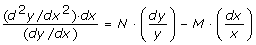(1)

Note that each term takes the fractional change form. It will be shown here, for N = M = 2, that this yields the second-order D.E., free of empirical constants, that gives the mathematical relation y = a·x/(b +x). This relation describes the saturation plot of Figure 1. Integration and analysis then lead to the definitions of the basic empirical constants that describe all saturation plots. Setting κ = dy/dx = slope gives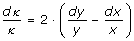(2)

where /κ is the fractional change in the slope.

Integrating and taking anti-logarithms gives the first-order D.E. for the slope,(3)

Integrating again and rearranging gives(4)

This algebraic relation, when substituted into equation (1), satisfies the second-order D.E. Therefore, it is a general solution. The system constants are determined by forcing the general solution to fit the physical boundary conditions (x → 0 and x → ∞), giving a unique solution.

Evaluate C1 and C2 using equation (4). Let x → 0, so that C1 >>C2·x, and therefore(5)

Rearranging so y = 1/[(C1/x) + C2], let x → ∞, then C2 = 1/y = 1/Υsat, where Υsat is the limiting value as y approaches the asymptote (saturation). Thus(6)

This equation defines the roles of the two directly measurable and independent empirical constants of the experimental system, κ0 and Ysat. Rearranging equation (6) gives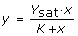(7)

the general form of the standard algebraic relation used to describe the data plot in Figure 1, [27, 912].

These saturation phenomena are typified by the binding of a substance (e.g., a ligand or substrate) to a binding site. This can be analyzed in terms of random interactions between x and the binding site. In general, yx/Υsat = Γbd, the fraction bound, which can be equated to the probability the site is occupied, for a given value of x. Thus Γbd = x/(K + x) = κ0·x/[Ysat + (κ0·x)]. The probability the site is free is Γfr = 1 - Γbd, so(8)

Thus, as x → 0, Γfr → 1, and as x → ∞, Γfr → 0.

Define Δ to mean the change in. Then the (change in slope)/(slope) equals Δ (dy/dx)/(dy/dx). Let Δ (dy/dx) = (1/2)·(d2y/dx2dx. The average slope is (y/x). Thus, Δ (y/x) = d (y/x), where d (y/x)/(y/x) = (dy/y) - (dx/x). Rearranging equation (1) with N = M = 2, and substituting equations (7) and (8) into it, yields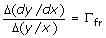(9)

Thus, the change in the slope (dy/dx) divided by the change in the average slope (y/x) is determined by Γfr.

Substituting into equation (3) for the slope gives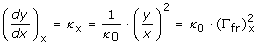(10)

### Ligand binding

Consider a small molecule, the ligand, that is present in either the free form, A, or the bound form, Ab. For the simplest case, assume that each ligand binds to a single specific binding site (bs). This could be on a macromolecule, Mbs, such as a protein. These sites are presumed to be independent and to have the same binding constant. The details of the experimental conditions required for these binding studies are found in standard reference texts [2, 5, 7, 912].

The basic overall binding reaction is defined to beThe necessary and sufficient condition for this analysis is the experimental data plot of Ab versus A. In Figure 1, set y = Ab and x = A. Then substitute into the key equations, for example, equation (6).(11)

The total number of binding sites in the experimental system (Mbs, A, Ab) is (Ab)sat. It is the limiting amount of ligand binding observed at saturation with A. The initial slope is κ0, the system's limiting binding rate when A → 0, and Γfr → 1. Thus, (Ab)sat and κ0 are the empirical constants of the ligand binding system. The conventional models of the binding mechanism identify Kd as the dissociation constant in mol L-1[2, 5, 6, 1012]. Equation (11) is often referred to as the Langmuir adsorption isotherm, or the Hill binding equation. It is sometimes written using the binding fraction, Γbd = Ab/(Ab)sat.

The units of κ0 = kbind·(Ab)sat, where kbind = 1/Kd, are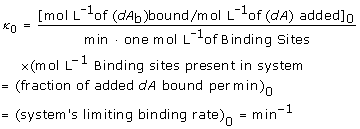Thus, kbind is the binding rate constant for one mole of binding sites evaluated at A → 0, where Γfr → 1. It characterizes the binding strength of the ligand for the binding site. Therefore, a high value of kbind means a high value of the initial slope of the system, κ0, and so the value of Kd is decreased.

The complete expression for the units of κ0 illustrates how descriptive information could be lost when units are cancelled. Thus, the units [mol L-1 of (dAb) bound/mol L-1 of (dA) added]0 describe a useful aspect of the binding process--the fraction of the added (dA) that is bound [(dAb)/dA], as A → 0. Taken over one minute, this yields the binding rate constant for one mole of binding sites.

The slope is given by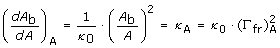(12)

where Γfr = (Ab)sat/[(Ab)sat + (κ0·A)]. Thus, κA is defined as the system's effective binding rate at any value of A--to distinguish it from the highest value of κ, when A → 0, giving κ0, the system's limiting binding rate. Thus, if κ0 is increased, then the ligand binding increases and (Γfr)A decreases, for a given value of A, because now more of the sites are occupied at A. If (Ab)sat is doubled, for example, Γfr will be increased--but not proportionately, see equation (11).

Knowing the value of κ0, obtained from experimental data, one can calculate Γfr for any A. Also,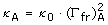, using equation (12), which shows how the slope depends on (1/A2). The values of κ0 and (Ab)sat can be obtained directly by using a standard linear transformation of the data plot, see the enzyme kinetics case, equation (19). This gives a plot of (A/Ab) versus A, with a slope of 1/(Ab)sat, and ordinate intercept of 1/κ0, see Figure 2.

### Other examples

The term, binding site, is used for convenience as a general way of identifying the interactive locus of many saturation phenomena. For example: ligand binds to a macromolecule; a nutrient molecule binds to a receptor on a bacterial membrane and is transported inside; a prey is bound to a predator's jaws; a substrate binds to an enzyme's catalytic site; a molecule is adsorbed at sites on a surface (Langmuir's adsorption). Some saturation phenomena are less well-suited to this binding site characterization--e.g., the stock-recruitment model for producing new fish biomass from spawning stock .

The simplest case of bacterial growth in a chemostat shows saturation dependence on the available nutrient [14, 15]. Thus, dr/dA = κA = (1/κ0)·(r/A)2 =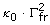, so(13a)

where r is the experimentally measured bacterial growth rate (g·L-1 min-1), at a given concentration of nutrient, A(g·L-1). Rsat is the limiting growth rate at saturation with nutrient (g·L-1 min-1). So, K = Rsat/κ0 in g·L-1, where the initial slope is κ0 (grams bacteria·L-1 min-1/grams nutrient·L-1), evaluated at A → 0. It measures the effectiveness of the specific bacteria's ability to convert a specific nutrient to bacterial growth--when all the receptor sites on the bacterial membrane are available. Thus, different bacteria using the same nutrient would have different values of κ0, reflecting the relative effectiveness of nutrient binding to the different receptor sites.

Consider predator-prey behavior in the simple case of the functional response model, where the attack rate increases, but at a decreasing rate with increased prey density . Here, dn/dA = κA = (1/κ0)·(n/A)2 =, so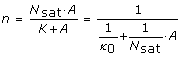(13b)

where n is the number of prey attacked over unit time by the predators present, and Nsat is the limiting rate of attack at saturation with prey. Set A equal to the prey density (e.g., number of prey per square kilometer). Then K = Nsat/κ0, where the initial slope, κ0, measures the effectiveness of the predator attacking the prey, as A → 0. Thus, a predator attacking two different prey yields different values of κ0.

### Michaelis-Menten (M-M) enzyme kinetics

The basic overall enzymatic reaction is the conversion of one substrate molecule, A, to one product molecule, P, by an enzyme molecule, E, that catalyzes this conversion at its catalytic site (cs).The necessary and sufficient condition for this analysis is the experimental data plot of (dP/dt) = p, versus A. See Figure 1, where p = y and A = x. The experimental conditions required for measuring p and A are described in standard reference texts [24, 7, 912]. The use here of p, instead of the conventional v, focuses attention on the actual measured quantity and how it relates to A, in terms of dp/dA and d2p/dA2.

Equation (9) becomes Δ (dp/dA)/Δ (p/A) = Γfr, and equation (1) gives(14)

Thus, the slope at any point, A, is κA = κ0·(Γfr)2, where, from equation (8), Γfr = Psat/[Psat + (κ0·A)]. Note that κA equals [(dA)converted/(dA)added]A = (dp/dA)A. This could be viewed as a measure of how effectively the system is converting substrate to product at A. It decreases rapidly as (1/A2).

Equation (6) becomes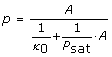(15)

The initial slope is κ0, and Psat is the limiting rate of enzyme catalysis when saturated with A. Increasing κ0 will increase the binding of A to the catalytic site. These are the two independent empirical constants of the experimental system (Et, A, P). Equating (Psat/κ0) to Km, the Michaelis constant, and with p = v, and Psat = V, gives(16)

the standard form for the M-M equation of enzyme kinetics.

Note that Psatkcat·Et, where Et is the total enzyme concentration present experimentally, which may not be known. The catalytic constant, kcat, is the limiting catalytic rate at which one mole of enzyme molecule could operate if completely saturated with substrate. Similarly, κ0kbind·Et, where kbind is here defined to be the binding rate constant of the substrate for the catalytic sites on one mole of enzyme (min-1 mol-1) -- evaluated when A → 0, where the catalytic site is maximally available, because Γfr → 1.

Equation (8) can be rewritten to give(17)

Thus, if kbind is increased, Γfr is decreased, because there are fewer free sites available at a given value of A. Whereas, if kcat is increased, Γfr is increased. The increased turnover rate means more free sites are available at a given value of A. As expected, Γfr is independent of Et, because Γfr depends only on the basic properties of the enzyme's catalytic function, kbind and kcat.

Enzyme kinetics differs from ligand binding because there is also a conversion step. The binding step is much faster than the conversion step, where the catalytic site converts the bound substrate to product and releases it. This is commonly assumed to involve a simple 1:1 stoichiometric relation between substrate bound and product released . The binding rate constant for one mole of enzyme is defined here to be = κ0/Et = kcat/Km. The ratio, kcat/Km, is often referred to as the specificity constant [19, 20]. Thus, kbind indicates the strength of the mutual interaction between a specific substrate and a specific enzyme, at the catalytic site, measured when A → 0. It defines a collective property for each particular combination of substrate and enzyme. For example, let A and Ecskbind, while A' and E'csk'bind, where kbind most probably differs from k'bind, but might not. Therefore, the higher the value of kbind, the more effectively does the substrate bind to the enzyme's catalytic site. The enzyme and substrate, taken together, perform better at higher values of kbind.

Thus, kbind and kcat can be considered the basic properties of this single enzyme molecule's catalytic function. So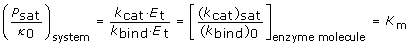(18)

Therefore, Km is defined by the ratio of the experimental system's empirical constants, which depend on the enzyme's basic properties. When Et is known, one can obtain values for kcat and kbind. Whereas, although Psat and κ0 often are measured experimentally where Et is not known, their ratio still gives Km. Doubling Et will double both Psat and κ0, so the ratio, Km, remains unchanged.

For clarity and convenience, the definitions and units of the various constants are explicitly stated here.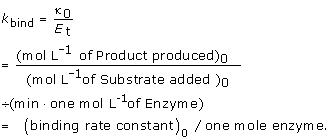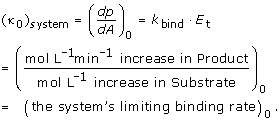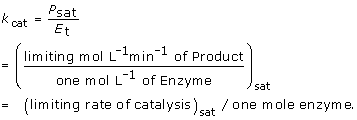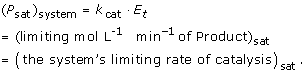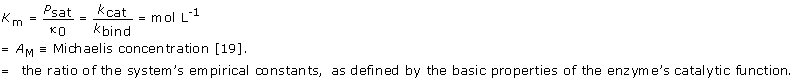There are various standard linear transformations of equations (15) and (16) that aid in the initial analysis of the data plot in Figure 1, . Equation (19) is one.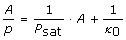(19)

This gives a linear plot of (A/p) versus A. The slope is (1/Psat) and the ordinate intercept is (1/κ0), recall Figure 2. This provides direct evaluation of the system's empirical constants, κ0, and Psat, from the experimental data. Using C1 = 1/κ0, obtained from equation (19), one can calculate κA, at any value of A, using the equation for κA.

## Discussion

### Basic case

The mathematical model presented here is based solely on the observed experimental data plot for these phenomena, as shown in Figure 1. This analysis of the second-order D.E. offers an alternative approach, free of mechanism, that describes the common process underlying all natural phenomena exhibiting saturation behavior. It provides a general mathematical description of these phenomena. The D.E. approach takes a path of discovery that reveals the salient features of these phenomena on the way to reaching y = x/[(1/κ0) + (1/Ysatx]. It complements approaches that model each specific saturation phenomenon separately, in terms of a proposed mechanism.

The D.E. analysis provided two general integration constants, C1 and C2, evaluated at the known boundary conditions, x → 0 and x → ∞. This gave the two empirical constants, κ0 and Ysat, that defined the relation between the variables of any saturation phenomenon -- see equation (6), the general algebraic description of these saturation phenomena. The empirical constant, κ0, the initial slope, and its practical significance, have not been recognized previously.

Applying the quantitative relation for Γfr clarified the functioning of the interactive site. It showed that the underlying relation describing these phenomena, equation (1), became Δ(dy/dx)/Δ(y/x) = Γfr, see equation (9). The slope, equation (3), became κ = κ0·(Γfr)2. Its strong dependence on (1/x2) was shown. As x increases, each added increment, dx, sees a lower Γfr, because a greater fraction of the sites are occupied at the instant of adding dx. This leaves fewer sites free to attend to the conversion of this additional dx. This behavior is the essence of how these saturation phenomena function in response to increased x.

### Ligand binding, bacterial growth, predator-prey

The response of these saturation phenomena to increased A is driven by Γfr, see equations (9) and (10). The independent empirical constants for each phenomenon relate the variables of each and define the K that characterizes each one, see equations (11), (13a) and (13b). This mathematical model defines K, in general, as the ratio of the limiting rate/initial slope. Figure 2 shows how to obtain their values from the data. Other applications of this general approach include surface adsorption, facilitated transport, and transmission of infection. It emphasizes the utility of the initial slope, κ0.

### Michaelis-Menten enzyme kinetics

Equation (10) shows that the slope, κA, depends on (Γfr)2 and (1/A2). Thus, Γfr drives the experimental system's behavior and accounts, quantitatively, for the decrease in the slope with increasing A. This leads to the concepts of:

• ■ the system's effective binding rate, for Et moles of enzyme, at A.

• ■ the binding rate constant for one mole of enzyme, at A → 0.

For Et moles, (dp/dA)0 = κ0, and for one mole, (dp/dA)0/Et = κ0/Et = kbind. Note that κA = (dp/dA)A can be calculated using the equation for the slope and equation (19). Thus, the (slope)A/(slope)0 = (Γfr)2, where Γfr = Psat/[Psat + (κ0·A)].

The D.E. analysis defined the two independent empirical constants of this experimental system as κ0 and Psat. Equation (15) is the general algebraic relation for illustrating their independent roles. Equation (18) ties together these empirical constants and the basic properties, kcat and kbind, to relate them to Km. Thus, kbind and kcat, taken together, can expand the ability to characterize and compare the interaction of enzymes and their substrates.

The usual model for the M-M enzyme reaction mechanism defines Km as a constant derived from the reaction rate constants. Such models are essential in pursuing the details of a proposed mechanism for M-M enzyme reactions, or for any saturation phenomenon. Yet, numerous different interpretations of what Km means have arisen in the literature, based on the standard model and mechanism. Some examples include: parameter, kinetic constant, not an independent kinetic constant, empirical quantity, a constant for the steady-state, measures affinity in the steady-state, should not be used as a measure of substrate affinity, most useful fundamental constant of enzyme chemistry, not a true equilibrium constant, dubious assertion that Km reflects an enzyme's affinity for its substrate [212, 21]. According to Riggs, "Notice that the Michaelis constant is not a rate constant, nor an affinity constant, nor a dissociation constant, but is merely a constant of convenience" . The interpretation presented here, based on the mathematical model, is rooted in equation (18). It showed that Km = kcat/kbind, the ratio of the enzyme's basic properties. Thus, this model viewed Km as a derived quantity, and not as an independent basic property of the enzyme molecule's catalytic function.

The action of enzyme inhibitors offers additional perspective on interpreting Km = kcat/kbind. Consider five basic cases of enzyme inhibition: Competitive, Uncompetitive, Pure Non-Competitive, Predominantly Competitive, Predominantly Uncompetitive . In no case does the inhibitor (I) cause the limiting rate, Psat, or the initial slope, κ0, of the observed data plot for the experimental system (Et, I, A, P) to increase. The value of Km = kcat/kbind, however, is observed to increase, remain unchanged, or decrease--depending on the relative effects of the inhibitor on kcat and kbind. Any basic property of an enzyme molecule's catalytic function should never increase in the presence of an inhibitor. Thus, kcat and kbind meet this condition. Their ratio, kcat/kbind = Km, does not. Therefore, Km is not one of the two basic properties. Changing from Km to AM, the Michaelis concentration, would be consistent with this interpretation .

The ratio of these observable empirical constants, Psat/κ0, defines Km. Thus, the mathematical analysis offers an operational definition of Km, independent of any interpretations . This approach to defining Km is consistent with all the known factors. "Definitions based on what is actually observed are therefore on a sounder and more lasting basis than those that depend on an assumed mechanism" . Numerous mechanisms can generate M-M kinetics; "Consequently there is no general definition of any of the kinetic parameters... in terms of the rate constants for the elementary steps of a reaction's mechanism" .

The algebraic relation, p = Psat·A/(Km + A), describes the data plot of an enzyme kinetic study. Its validity is independent of any mechanism. The mechanism-free approach derives this algebraic relation directly from the second-order D.E. This general analysis also reveals the underlying factors, such as Γfr, that govern the basic behavior of these saturation phenomena.

The enzyme's catalytic function involves two distinct processes, binding the substrate and converting it to product. This mathematical analysis demonstrated that the two empirical constants of the D.E., κ0 = Et·kbind and Psat = Et·kcat, define these two processes--binding and catalysis--in terms of the basic properties, kbind and kcat.

## Conclusions

The results presented here are completely general and based entirely on a mathematical model that analyzes the observed experimental data plot for the relation between the independent and dependent variables. They apply directly to every natural phenomenon displaying the characteristic saturation behavior that produces the hyperbolic kinetics described by the relation,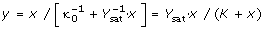. The second-order D.E. presented here reveals the basic underlying relation that applies to these phenomena and its dependence on the probability a site is free. The analysis provides a theoretical basis for defining the empirical constants and basic properties of each saturation phenomenon, based on the two constants of integration evaluated at the boundary conditions. The universality of these saturation phenomena makes it useful to have the D.E., free of constants, that describes the basic properties of all these systems.

The first-order D.E. derived here introduces the concept of the effective binding rate. It is directly related to the slope of the experimental data plot. The initial slope is where it is highest, the binding rate constant of the ligand for the binding site. The analysis revealed the significance of the initial slope as an independent empirical constant for these systems exhibiting saturation behavior, and its role in determining the probability that the active site is free.

## References

1. Mellor J: Higher Mathematics for Students of Chemistry and Physics. 1955, New York: Dover

2. Bisswanger H: Enzyme Kinetics. 2008, Weinheim: Wiley-VCH, 2

3. Cook P, Cleland W: Enzyme Kinetics and Mechanism. 2007, New York: Garland Science

4. Cornish-Bowden A, Wharton C: Enzyme Kinetics. 1988, Oxford: IRL Press

5. Klotz I: Ligand-Receptor Energetics. 1997, New York: Wiley

6. Matthews J: Fundamentals of Receptor, Enzyme and Transport Kinetics. 1993, London: CRC

7. Purich D, Allison R: Handbook of Biochemical Kinetics. 2000, New York: Academic Press

8. Hammes G: Physical Chemistry for the Biological Sciences. 2007, New York: Wiley-Interscience

9. Bisswanger H: Practical Enzymology. 2004, Weinheim: Wiley-VCH

10. Gutfreund H: Kinetics for the Life Sciences. 1995, Cambridge University Press

11. Van Holde K, Johnson W, Ito P: Principles of Physical Biochemistry. 2006, New Jersey: Pearson/Prentice Hall, 2

12. Wilson K, Walker J: Practical Biochemistry. 2000, Cambridge University Press, 5

13. Beverton R, Holt S: On the dynamics of exploited fish populations. 1959, London: HMSO

14. Monod J: La Technique de culture continue: theorie et applications. Ann Inst Pasteur. 1950, 79: 390-410.

15. Edelstein-Keshet L: Mathematical models in biology. 1988, New York: Random House

16. Holling CS: The components of predation as revealed by a study of small mammal predation of the European pine saw fly. Can Entomol. 1959, 91: 293-320. 10.4039/Ent91293-5.

17. Real L: The kinetics of functional response. Am Naturalist. 1977, 111: 289-300. 10.1086/283161.

18. Berryman A: The origin and evolution of predator-prey theory. Ecology. 1992, 73: 1530-1535. 10.2307/1940005.

19. International Union of Biochemistry: Symbolism and terminology in enzyme kinetics. Biochem J. 1983, 213: 561-571.

20. Koshland DE: The application and usefulness of the ratio kcat/K(M). Bioorg Chem. 2002, 30: 211-213. 10.1006/bioo.2002.1246.

21. Netter H: Theoretical Biochemistry. 1969, Edinburgh: Oliver and Boyd

22. Riggs D: The Mathematical Approach to Physiological Problems. 1963, Cambridge: MIT Press

## Author information

Authors

### Corresponding author

Correspondence to Gordon R Kepner.

### Competing interests

The author declares that he has no competing interests.

## Authors’ original submitted files for images

Below are the links to the authors’ original submitted files for images.

## Rights and permissions

Reprints and Permissions

Kepner, G.R. Saturation Behavior: a general relationship described by a simple second-order differential equation. Theor Biol Med Model 7, 11 (2010). https://doi.org/10.1186/1742-4682-7-11

• Accepted:

• Published:

• DOI: https://doi.org/10.1186/1742-4682-7-11

### Keywords

• Initial Slope
• Enzyme Kinetic
• Empirical Constant
• Data Plot
• Algebraic Relation